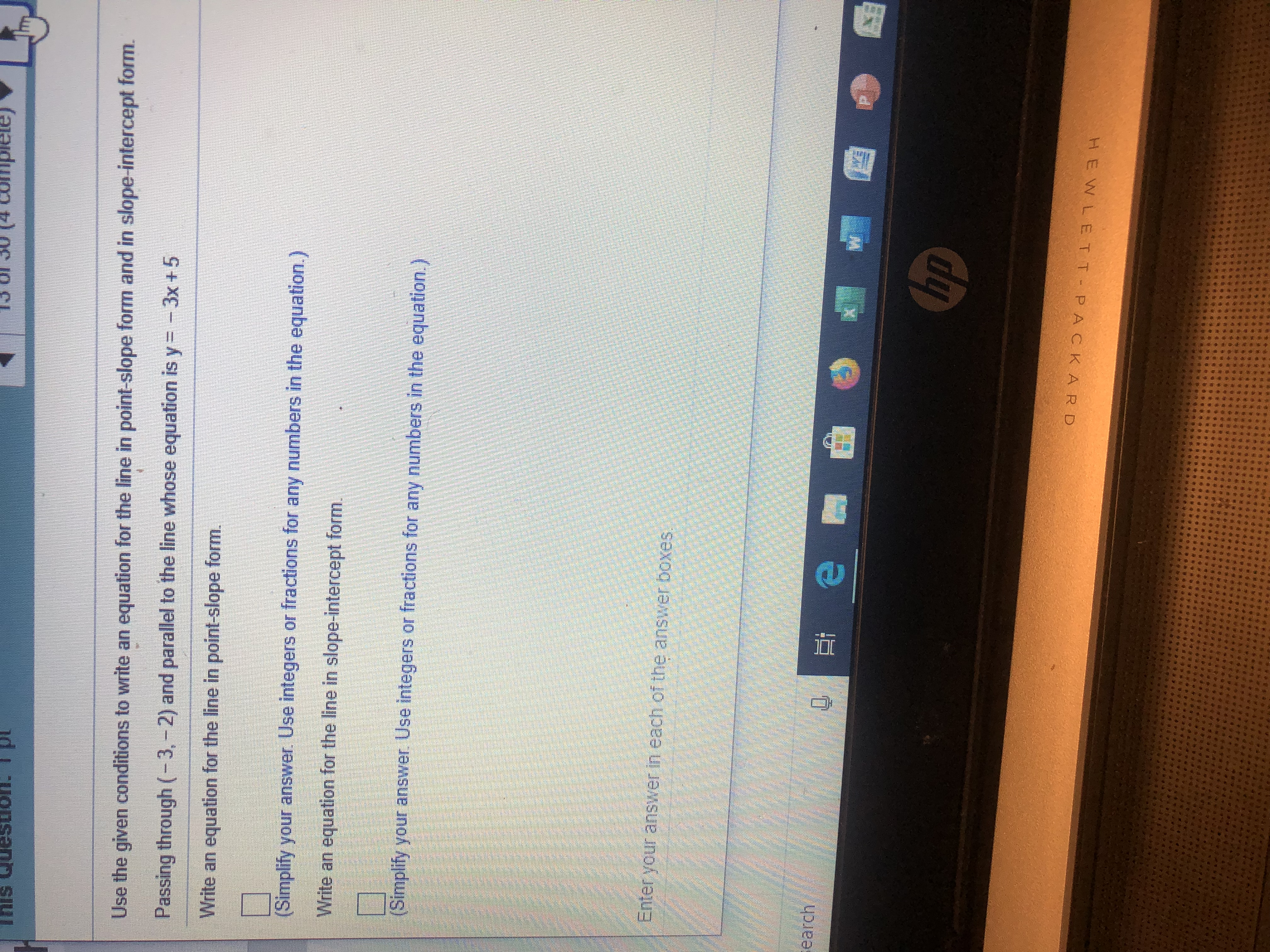mplele)(4ptUse the given conditions to write an equation for the line in point-slope form and in slope-intercept form.Passing through (-3, -2) and parallel to the line whose equation is y= -3x +5Write an equation for the line in point-slope form.(Simplify your answer. Use integers or fractions for any numbers in the equation.)Write an equation for the line in slope-intercept form.(Simplify your answer Use integers or fractions for any numbers in the equation.)enter your answer in each of the answer boxesearchehpHEWLE T TPAC K ARD

Questionhelp_outlineImage Transcriptionclosemplele) (4 pt Use the given conditions to write an equation for the line in point-slope form and in slope-intercept form. Passing through (-3, -2) and parallel to the line whose equation is y= -3x +5 Write an equation for the line in point-slope form. (Simplify your answer. Use integers or fractions for any numbers in the equation.) Write an equation for the line in slope-intercept form. (Simplify your answer Use integers or fractions for any numbers in the equation.) enter your answer in each of the answer boxes earch e hp HEWLE T TPAC K ARD fullscreen
Step 1

Given, Required line passing through the point (-3 , -2) and parallel to the line y = -3x+5.

Step 2

Slope of the given line is, m = -3

Since the parallel lines have same slope, therefore slope of required line is also -3.

Step 3

So point slope of the required...

Want to see the full answer?

See Solution

Want to see this answer and more?

Our solutions are written by experts, many with advanced degrees, and available 24/7

See Solution
Tagged in

Other